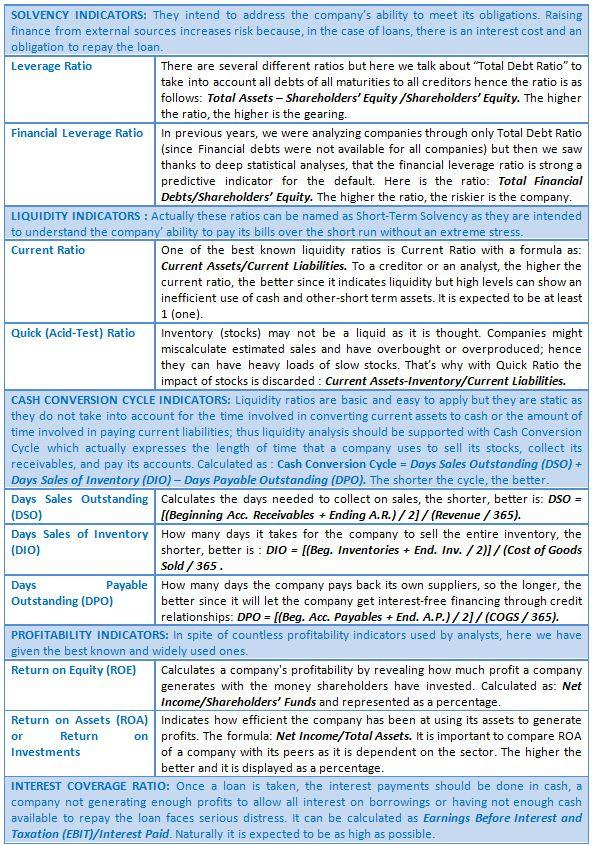• ITA
Category: Credit Rating Methodologies

# Financial Ratio Analysis for Dummies :) [Part 1]

Following our the most-read “Fast and furious Credit Risk Check” post, we have thought that we could address this topic again with some further explanations.
Here, we have prepared a very practical guide for the most well-known financial ratios which you can simply print and post it on your wall.

### SOLVENCY INDICATORS:

They intend to address the company’s ability to meet its obligations. Raising finance from external sources increases risk because, in the case of loans, there is an interest cost and an obligation to repay the loan.

Leverage Ratio : There are several different ratios but here we talk about “Total Debt Ratio” to take into account all debts of all maturities to all creditors hence the ratio is as follows: Total Assets – Shareholders’ Equity /Shareholders’ Equity. The higher the ratio, the higher is the gearing.

Financial Leverage Ratio : In previous years, we were analyzing companies through only Total Debt Ratio (since Financial debts were not available for all companies) but then we saw thanks to deep statistical analyses, that the financial leverage ratio is strong a predictive indicator for the default. Here is the ratio: Total Financial Debts/Shareholders’ Equity. The higher the ratio, the riskier is the company.

### LIQUIDITY INDICATORS:

Actually these ratios can be named as Short-Term Solvency as they are intended to understand the company’ ability to pay its bills over the short run without an extreme stress. Current Ratio: One of the best known liquidity ratios is Current Ratio with a formula as: Current Assets/Current Liabilities. To a creditor or an analyst, the higher the current ratio, the better since it indicates liquidity but high levels can show an inefficient use of cash and other-short term assets. It is expected to be at least 1 (one).

Quick (Acid-Test) Ratio: Inventory (stocks) may not be a liquid as it is thought. Companies might miscalculate estimated sales and have overbought or overproduced; hence they can have heavy loads of slow stocks. That’s why with Quick Ratio the impact of stocks is discarded : Current Assets-Inventory/Current Liabilities.

### CASH CONVERSION CYCLE INDICATORS:

Liquidity ratios are basic and easy to apply but they are static as they do not take into account for the time involved in converting current assets to cash or the amount of time involved in paying current liabilities; thus liquidity analysis should be supported with Cash Conversion Cycle which actually expresses the length of time that a company uses to sell its stocks, collect its receivables, and pay its accounts. Calculated as : Cash Conversion Cycle = Days Sales Outstanding (DSO) + Days Sales of Inventory (DIO) – Days Payable Outstanding (DPO).The shorter the cycle, the better.

Days Sales Outstanding (DSO): Calculates the days needed to collect on sales, the shorter, better is: DSO = [(Beginning Acc. Receivables + Ending A.R.) / 2] / (Revenue / 365).

Days Sales of Inventory (DIO): How many days it takes for the company to sell the entire inventory, the shorter, better is : DIO = [(Beg. Inventories + End. Inv. / 2)] / (Cost of Goods Sold / 365) .

Days Payable Outstanding (DPO): How many days the company pays back its own suppliers, so the longer, the better since it will let the company get interest-free financing through credit relationships: DPO = [(Beg. Acc. Payables + End. A.P.) / 2] / (COGS / 365).

### PROFITABILITY INDICATORS:

In spite of countless profitability indicators used by analysts, here we have given the best known and widely used ones.

Return on Equity (ROE): Calculates a company’s profitability by revealing how much profit a company generates with the money shareholders have invested. Calculated as: Net Income/Shareholders’ Funds and represented as a percentage.

Return on Assets (ROA) or Return on Investments: Indicates how efficient the company has been at using its assets to generate profits. The formula: Net Income/Total Assets. It is important to compare ROA of a company with its peers as it is dependent on the sector. The higher the better and it is displayed as a percentage.

### INTEREST COVERAGE RATIO:

Once a loan is taken, the interest payments should be done in cash, a company not generating enough profits to allow all interest on borrowings or having not enough cash available to repay the loan faces serious distress. It can be calculated as Earnings Before Interest and Taxation(EBIT)/Interest Paid. Naturally it is expected to be as high as possible. Here below, we also provide you a table of all the ratios we explained. We hope it helps you!Financial Ratio Analysis Guide